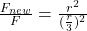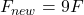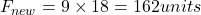## Objects 1 and 2 attract each other with a electrostatic force of 18.0 units. If the distance separating Objects 1 and 2 is changed to one-th

Question

Objects 1 and 2 attract each other with a electrostatic force of 18.0 units. If the distance separating Objects 1 and 2 is changed to one-third the original value, then the new electrostatic force will be _____ units.

in progress 0
3 months 2021-07-26T01:46:24+00:00 1 Answers 2 views 0

New force will become 162 units

Explanation:

Let the charge on each object is q and distance between then is r

Electrostatic force between to object is given byFrom the relation we can see that electrostatic force is inversely proportional to square of distance between them

Therefore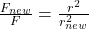Now  new distance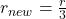So